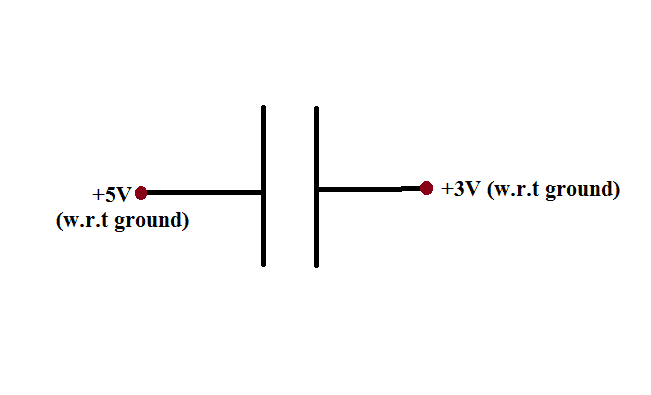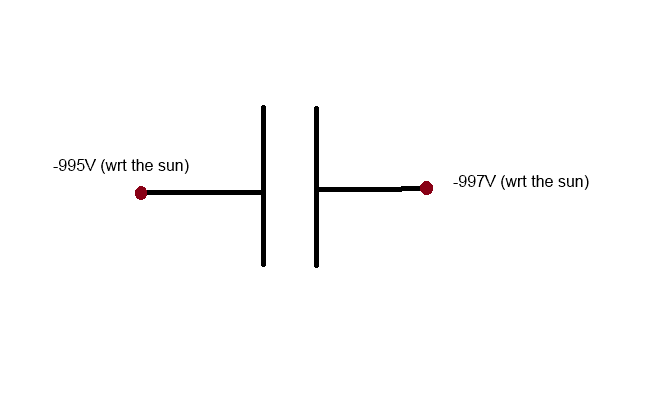# Both plates of capacitor connected to positve voltage

If both the plates of a capacitor are connected to positve voltage, (but has a potential differnce between them) will the capacitor be charged? And how?Bystander
Homework Helper
Gold Member
And what potential is this particular ground at with respect to another ground? Does the ground potential matter?

And what potential is this particular ground at with respect to another ground? Does the ground potential matter?
Actually, by 'ground', I mean something which is electrically neutral. Both of the plates are connected to some conductor which has lack of electrons (and so they are electrically positive). But the voltages of the plates are not the same.

Drakkith
Staff Emeritus
Well, if one plate is charged to +5v and the other to +3v, is there a potential difference between them?

Well, if one plate is charged to +5v and the other to +3v, is there a potential difference between them?
Why not? It's $(+5V) - (+3V) = 2V$

Drakkith
Staff Emeritus
Okay. So if the potential between the plates is 2 volts, do you think the capacitor is "charged"? Why or why not?

Okay. So if the potential between the plates is 2 volts, do you think the capacitor is "charged"? Why or why not?
In a capacitor, one of the plates is charged positive and the other negative and they are equal in quantity. But in this case, both the plates lack electron. So how can it provide negative charge or, electron?
If I assume, the plate connected to $3V$ is charged negative, then the plate will have a negative electric potential, and so there should be a current flow because it is connected with a voltage generator which will always keep potential to $+3V$.

Last edited:
Drakkith
Staff Emeritus
Both plates lack a very, very small number of electrons. Over 99.999% of the electrons are still on the plates. The plate charged to +5v simply has a few more electrons missing. A few missing electrons adds up to a large amount of charge.

Both plates lack a very, very small number of electrons. Over 99.999% of the electrons are still on the plates. The plate charged to +5v simply has a few more electrons missing. A few missing electrons adds up to a large amount of charge.

But the point is, if the plate connected to $+3V$ is negatively charged, then it will have a negative electric potential. As, it is connected to a voltage generator which always keeps the voltage to $+3V$, there should be a current flow. So, the plate will keep losing electrons, won't it?

Drakkith
Staff Emeritus
Not at all. The plate will lose electrons until it reaches +3 volts. It will then stop losing them and remain at +3v.

Not at all. The plate will lose electrons until it reaches +3 volts. It will then stop losing them and remain at +3v.
How can the plate have negative charge, but positive electric potential?

Drakkith
Staff Emeritus
How can the plate have negative charge, but positive electric potential?

It doesn't have a "negative charge", it has -2 volts of potential relative to ground compared to the +5v plate. Remember that electric potential is measured between two points. Measuring the voltage between the ground and each plate gives you +3v and +5v, but measuring from one plate to the other gives you either +2v or -2v. In other words, if you measure the voltage between the plates with a volt meter, you'll get either +2v or -2v depending on how you place the leads. Switching them around will cause current to flow the other way through the voltmeter and it will read the voltage a being the opposite sign.

It doesn't have a "negative charge", it has -2 volts of potential relative to ground compared to the +5v plate. Remember that electric potential is measured between two points. Measuring the voltage between the ground and each plate gives you +3v and +5v, but measuring from one plate to the other gives you either +2v or -2v. In other words, if you measure the voltage between the plates with a volt meter, you'll get either +2v or -2v depending on how you place the leads. Switching them around will cause current to flow the other way through the voltmeter and it will read the voltage a being the opposite sign.
So, you are saying, both the plates will have positive charges?

Drakkith
Staff Emeritus
So, you are saying, both the plates will have positive charges?

Relative to ground, yes.

anorlunda
Staff Emeritus
arpon,

Absolute potentials don't matter, only relative potentials. You could label both plates as +5 +3 or +1005 +1003 or -1005 -1003, and the result is the same.

Zero volts, is an arbitrary choice. We normally choose "ground" as zero volts becasuse it is natural and convenient. But other choices for zero work just as well.

An "uncharged object" has the same number of electrons as protons. But even an uncharged object can have a plus or minus potential difference between it and some other object.

Think relative, not absolute voltage. Balls roll down a ramp the same if the ramp is at the top of a mountain or at the bottom of a valley, as long as one end of the ramp is at a height higher relative to the other end.

CWatters
Homework Helper
Gold Member
Stop press. Scientists discover the earth isn't at 0V. The "ground" we have all being relying on is actually at -1000V wrt the sun today. Would it make any difference to your circuit?In the typical situation (which is most commonly described in text books), one of the plate is charged $+Q$ and the other $-Q$; If the potential difference is $V$, then the capacitance is defined as $C = \frac{Q}{V}$.
Then, what it would be in this case as I mentioned in post #1. My question is in the point that Drakkith said both the charges are positive.
arpon,

Absolute potentials don't matter, only relative potentials. You could label both plates as +5 +3 or +1005 +1003 or -1005 -1003, and the result is the same.

Zero volts, is an arbitrary choice. We normally choose "ground" as zero volts becasuse it is natural and convenient. But other choices for zero work just as well.

An "uncharged object" has the same number of electrons as protons. But even an uncharged object can have a plus or minus potential difference between it and some other object.

Think relative, not absolute voltage. Balls roll down a ramp the same if the ramp is at the top of a mountain or at the bottom of a valley, as long as one end of the ramp is at a height higher relative to the other end.
Relative to ground, yes.

anorlunda
Staff Emeritus
Relative to ground yes, but also relative to any common point (of which ground is a convenient but not mandatory point).

Look at all the responses so far. They are all telling you the same thing.

Drakkith
Staff Emeritus
In the typical situation (which is most commonly described in text books), one of the plate is charged $+Q$ and the other $-Q$; If the potential difference is $V$, then the capacitance is defined as $C = \frac{Q}{V}$.